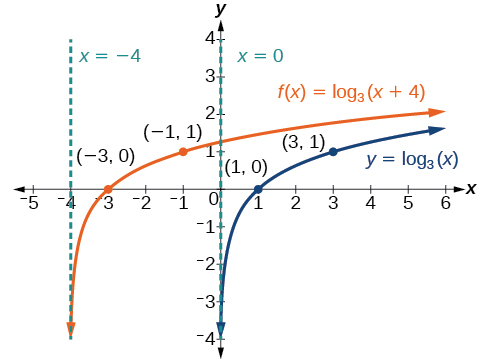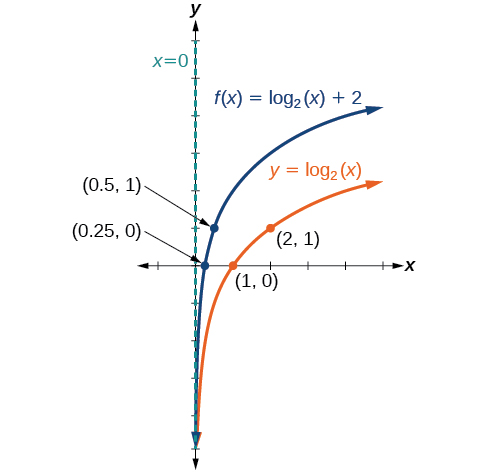# 6.4 Graphs of logarithmic functions  (Page 4/8)

 Page 4 / 8

Sketch a graph of $\text{\hspace{0.17em}}f\left(x\right)={\mathrm{log}}_{3}\left(x+4\right)\text{\hspace{0.17em}}$ alongside its parent function. Include the key points and asymptotes on the graph. State the domain, range, and asymptote.The domain is $\text{\hspace{0.17em}}\left(-4,\infty \right),$ the range $\text{\hspace{0.17em}}\left(-\infty ,\infty \right),$ and the asymptote $\text{\hspace{0.17em}}x=–4.$

## Graphing a vertical shift of y = log b ( x )

When a constant $\text{\hspace{0.17em}}d\text{\hspace{0.17em}}$ is added to the parent function $\text{\hspace{0.17em}}f\left(x\right)={\mathrm{log}}_{b}\left(x\right),$ the result is a vertical shift     $\text{\hspace{0.17em}}d\text{\hspace{0.17em}}$ units in the direction of the sign on $\text{\hspace{0.17em}}d.\text{\hspace{0.17em}}$ To visualize vertical shifts, we can observe the general graph of the parent function $\text{\hspace{0.17em}}f\left(x\right)={\mathrm{log}}_{b}\left(x\right)\text{\hspace{0.17em}}$ alongside the shift up, $\text{\hspace{0.17em}}g\left(x\right)={\mathrm{log}}_{b}\left(x\right)+d\text{\hspace{0.17em}}$ and the shift down, $\text{\hspace{0.17em}}h\left(x\right)={\mathrm{log}}_{b}\left(x\right)-d.$ See [link] .

## Vertical shifts of the parent function y = log b ( x )

For any constant $\text{\hspace{0.17em}}d,$ the function $\text{\hspace{0.17em}}f\left(x\right)={\mathrm{log}}_{b}\left(x\right)+d$

• shifts the parent function $\text{\hspace{0.17em}}y={\mathrm{log}}_{b}\left(x\right)\text{\hspace{0.17em}}$ up $\text{\hspace{0.17em}}d\text{\hspace{0.17em}}$ units if $\text{\hspace{0.17em}}d>0.$
• shifts the parent function $\text{\hspace{0.17em}}y={\mathrm{log}}_{b}\left(x\right)\text{\hspace{0.17em}}$ down $\text{\hspace{0.17em}}d\text{\hspace{0.17em}}$ units if $\text{\hspace{0.17em}}d<0.$
• has the vertical asymptote $\text{\hspace{0.17em}}x=0.$
• has domain $\text{\hspace{0.17em}}\left(0,\infty \right).$
• has range $\text{\hspace{0.17em}}\left(-\infty ,\infty \right).$

Given a logarithmic function with the form $\text{\hspace{0.17em}}f\left(x\right)={\mathrm{log}}_{b}\left(x\right)+d,$ graph the translation.

1. Identify the vertical shift:
• If $\text{\hspace{0.17em}}d>0,$ shift the graph of $\text{\hspace{0.17em}}f\left(x\right)={\mathrm{log}}_{b}\left(x\right)\text{\hspace{0.17em}}$ up $\text{\hspace{0.17em}}d\text{\hspace{0.17em}}$ units.
• If $\text{\hspace{0.17em}}d<0,$ shift the graph of $\text{\hspace{0.17em}}f\left(x\right)={\mathrm{log}}_{b}\left(x\right)$ down $\text{\hspace{0.17em}}d\text{\hspace{0.17em}}$ units.
2. Draw the vertical asymptote $\text{\hspace{0.17em}}x=0.$
3. Identify three key points from the parent function. Find new coordinates for the shifted functions by adding $\text{\hspace{0.17em}}d\text{\hspace{0.17em}}$ to the $\text{\hspace{0.17em}}y\text{\hspace{0.17em}}$ coordinate.
4. Label the three points.
5. The domain is $\text{\hspace{0.17em}}\left(0,\infty \right),$ the range is $\text{\hspace{0.17em}}\left(-\infty ,\infty \right),$ and the vertical asymptote is $\text{\hspace{0.17em}}x=0.$

## Graphing a vertical shift of the parent function y = log b ( x )

Sketch a graph of $\text{\hspace{0.17em}}f\left(x\right)={\mathrm{log}}_{3}\left(x\right)-2\text{\hspace{0.17em}}$ alongside its parent function. Include the key points and asymptote on the graph. State the domain, range, and asymptote.

Since the function is $\text{\hspace{0.17em}}f\left(x\right)={\mathrm{log}}_{3}\left(x\right)-2,$ we will notice $\text{\hspace{0.17em}}d=–2.\text{\hspace{0.17em}}$ Thus $\text{\hspace{0.17em}}d<0.$

This means we will shift the function $\text{\hspace{0.17em}}f\left(x\right)={\mathrm{log}}_{3}\left(x\right)\text{\hspace{0.17em}}$ down 2 units.

The vertical asymptote is $\text{\hspace{0.17em}}x=0.$

Consider the three key points from the parent function, $\text{\hspace{0.17em}}\left(\frac{1}{3},-1\right),$ $\left(1,0\right),$ and $\text{\hspace{0.17em}}\left(3,1\right).$

The new coordinates are found by subtracting 2 from the y coordinates.

Label the points $\text{\hspace{0.17em}}\left(\frac{1}{3},-3\right),$ $\left(1,-2\right),$ and $\text{\hspace{0.17em}}\left(3,-1\right).$

The domain is $\text{\hspace{0.17em}}\left(0,\infty \right),$ the range is $\text{\hspace{0.17em}}\left(-\infty ,\infty \right),$ and the vertical asymptote is $\text{\hspace{0.17em}}x=0.$

The domain is $\text{\hspace{0.17em}}\left(0,\infty \right),$ the range is $\text{\hspace{0.17em}}\left(-\infty ,\infty \right),$ and the vertical asymptote is $\text{\hspace{0.17em}}x=0.$

Sketch a graph of $\text{\hspace{0.17em}}f\left(x\right)={\mathrm{log}}_{2}\left(x\right)+2\text{\hspace{0.17em}}$ alongside its parent function. Include the key points and asymptote on the graph. State the domain, range, and asymptote.The domain is $\text{\hspace{0.17em}}\left(0,\infty \right),$ the range is $\text{\hspace{0.17em}}\left(-\infty ,\infty \right),$ and the vertical asymptote is $\text{\hspace{0.17em}}x=0.$

## Graphing stretches and compressions of y = log b ( x )

When the parent function $\text{\hspace{0.17em}}f\left(x\right)={\mathrm{log}}_{b}\left(x\right)\text{\hspace{0.17em}}$ is multiplied by a constant $\text{\hspace{0.17em}}a>0,$ the result is a vertical stretch    or compression of the original graph. To visualize stretches and compressions, we set $\text{\hspace{0.17em}}a>1\text{\hspace{0.17em}}$ and observe the general graph of the parent function $\text{\hspace{0.17em}}f\left(x\right)={\mathrm{log}}_{b}\left(x\right)\text{\hspace{0.17em}}$ alongside the vertical stretch, $\text{\hspace{0.17em}}g\left(x\right)=a{\mathrm{log}}_{b}\left(x\right)\text{\hspace{0.17em}}$ and the vertical compression, $\text{\hspace{0.17em}}h\left(x\right)=\frac{1}{a}{\mathrm{log}}_{b}\left(x\right).$ See [link] .

## Vertical stretches and compressions of the parent function y = log b ( x )

For any constant $\text{\hspace{0.17em}}a>1,$ the function $\text{\hspace{0.17em}}f\left(x\right)=a{\mathrm{log}}_{b}\left(x\right)$

• stretches the parent function $\text{\hspace{0.17em}}y={\mathrm{log}}_{b}\left(x\right)\text{\hspace{0.17em}}$ vertically by a factor of $\text{\hspace{0.17em}}a\text{\hspace{0.17em}}$ if $\text{\hspace{0.17em}}a>1.$
• compresses the parent function $\text{\hspace{0.17em}}y={\mathrm{log}}_{b}\left(x\right)\text{\hspace{0.17em}}$ vertically by a factor of $\text{\hspace{0.17em}}a\text{\hspace{0.17em}}$ if $\text{\hspace{0.17em}}0
• has the vertical asymptote $\text{\hspace{0.17em}}x=0.$
• has the x -intercept $\text{\hspace{0.17em}}\left(1,0\right).$
• has domain $\text{\hspace{0.17em}}\left(0,\infty \right).$
• has range $\text{\hspace{0.17em}}\left(-\infty ,\infty \right).$

bsc F. y algebra and trigonometry pepper 2
given that x= 3/5 find sin 3x
4
DB
remove any signs and collect terms of -2(8a-3b-c)
-16a+6b+2c
Will
Joeval
(x2-2x+8)-4(x2-3x+5)
sorry
Miranda
x²-2x+9-4x²+12x-20 -3x²+10x+11
Miranda
x²-2x+9-4x²+12x-20 -3x²+10x+11
Miranda
(X2-2X+8)-4(X2-3X+5)=0 ?
master
The anwser is imaginary number if you want to know The anwser of the expression you must arrange The expression and use quadratic formula To find the answer
master
The anwser is imaginary number if you want to know The anwser of the expression you must arrange The expression and use quadratic formula To find the answer
master
Y
master
master
Soo sorry (5±Root11* i)/3
master
Mukhtar
explain and give four example of hyperbolic function
What is the correct rational algebraic expression of the given "a fraction whose denominator is 10 more than the numerator y?
y/y+10
Mr
Find nth derivative of eax sin (bx + c).
Find area common to the parabola y2 = 4ax and x2 = 4ay.
Anurag
A rectangular garden is 25ft wide. if its area is 1125ft, what is the length of the garden
to find the length I divide the area by the wide wich means 1125ft/25ft=45
Miranda
thanks
Jhovie
What do you call a relation where each element in the domain is related to only one value in the range by some rules?
A banana.
Yaona
given 4cot thither +3=0and 0°<thither <180° use a sketch to determine the value of the following a)cos thither
what are you up to?
nothing up todat yet
Miranda
hi
jai
hello
jai
Miranda Drice
jai
aap konsi country se ho
jai
which language is that
Miranda
I am living in india
jai
good
Miranda
what is the formula for calculating algebraic
I think the formula for calculating algebraic is the statement of the equality of two expression stimulate by a set of addition, multiplication, soustraction, division, raising to a power and extraction of Root. U believe by having those in the equation you will be in measure to calculate it
Miranda
state and prove Cayley hamilton therom
hello
Propessor
hi
Miranda
the Cayley hamilton Theorem state if A is a square matrix and if f(x) is its characterics polynomial then f(x)=0 in another ways evey square matrix is a root of its chatacteristics polynomial.
Miranda
hi
jai
hi Miranda
jai
thanks
Propessor
welcome
jai
What is algebra
algebra is a branch of the mathematics to calculate expressions follow.
Miranda
Miranda Drice would you mind teaching me mathematics? I think you are really good at math. I'm not good at it. In fact I hate it. 😅😅😅
Jeffrey
lolll who told you I'm good at it
Miranda
something seems to wispher me to my ear that u are good at it. lol
Jeffrey
lolllll if you say so
Miranda
but seriously, Im really bad at math. And I hate it. But you see, I downloaded this app two months ago hoping to master it.
Jeffrey
which grade are you in though
Miranda
oh woww I understand
Miranda
Jeffrey
Jeffrey
Miranda
how come you finished in college and you don't like math though
Miranda
gotta practice, holmie
Steve
if you never use it you won't be able to appreciate it
Steve
I don't know why. But Im trying to like it.
Jeffrey
yes steve. you're right
Jeffrey
so you better
Miranda
what is the solution of the given equation?
which equation
Miranda
I dont know. lol
Jeffrey
Miranda
JeffreyByByBy Heather McAvoyBy Stephen VoronBy Brooke DelaneyBy Frank LevyBy Stephen VoronBy OpenStaxBy Madison ChristianBy Vanessa SoledadBy Michael NelsonBy Richley Crapo